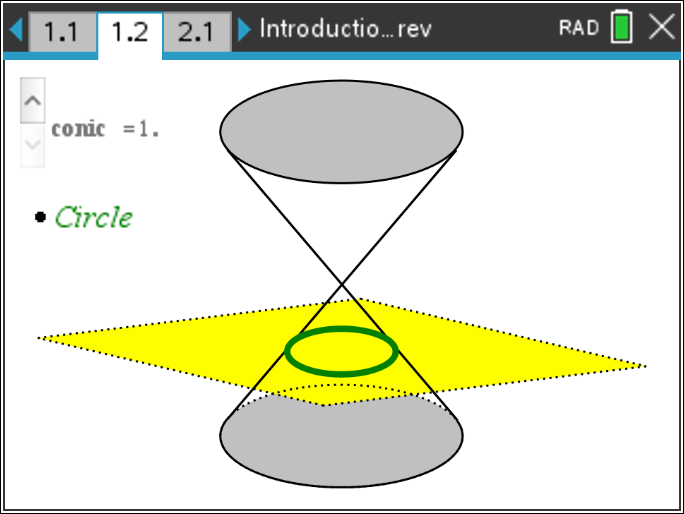# Activities

•##### Download

•• ##### Subject Area

• Math: Precalculus: Analytic Geometry (Conics)

• ##### Author9-12

45 Minutes

• ##### Device
•TI-Nspire™ CX/CX II
•TI-Nspire™ CX CAS/CX II CAS
• TI-Nspire™ Navigator™
• TI-Nspire™ Apps for iPad®
• ##### Software

TI-Nspire™ CX
TI-Nspire™ CX CAS

5.0

• ##### Report an Issue

Introduction to Conic Sections

Updated on 05/06/2019#### Activity Overview

This lesson involves observing how each of the conic sections is formed and connecting the locus definition of a parabola with the vertex form of a parabola.

#### Objectives

• Students will identify how each conic results from slicing cones.
• Students will understand the locus definition of a parabola.
• Students will describe how the values of a, h, and k in the vertex form of the equation of a parabola affect its graph.
• Students will use the locus definition of a parabola to derive the equation of a parabola and will describe the relationships among the focus, the directrix, and the values in the vertex form of a parabola.

#### Vocabulary

• circle
• ellipse
• parabola
• hyperbola
• axis of symmetry
• focus
• directrix

#### About the Lesson

This lesson involves observing how each of the conic sections is formed and connecting the locus definition of a parabola with the vertex form of a parabola.
As a result, students will:

• Explain how each of the conic sections is formed.
• Manipulate a point on a parabola and the focus of a parabola to discover the locus definition.
• Manipulate a, h, and k in the vertex form of a parabola to observe the effects of each value.
• Use the locus definition to derive the equation of a parabola given the focus, directrix, and any point on the parabola.
• Identify the relationships among the values of the vertex form of a parabola and the focus.